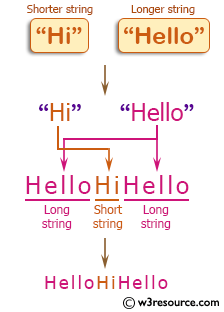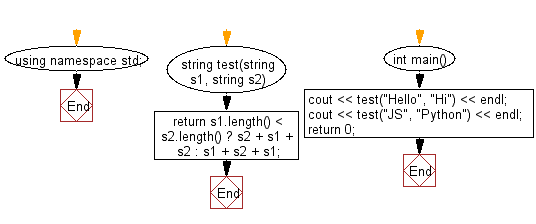﻿ C++ : New string from two strings, long + short + long# C++ Exercises: Create a new string from two given string one is shorter and another is longer

## C++ Basic Algorithm: Exercise-63 with Solution

Write a C++ program to create a new string from two given string one is shorter and another is longer. The format of the new string will be long string + short string + long string.

Sample Solution:

C++ Code :

``````#include <iostream>
using namespace std;

string test(string s1, string s2)
{
return s1.length() < s2.length() ? s2 + s1 + s2 : s1 + s2 + s1;
}

int main()
{
cout << test("Hello", "Hi") << endl;
cout << test("JS", "Python") << endl;
return 0;
}
``````

Sample Output:

```HelloHiHello
PythonJSPython
```

Pictorial Presentation:Flowchart:C++ Code Editor:

Contribute your code and comments through Disqus.

What is the difficulty level of this exercise?

﻿